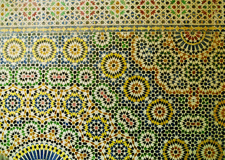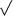# issue contentsFOUNDATIONSADVANCES
ISSN: 2053-2733

# September 2008 issueCover illustration: An octagonal mosaic from the Kasbah de Telouet, High Atlas, Morocco, based on a modified quasilattice. The underlying quasilattice can best be seen in the blue-and-black portions of the pattern where the `standing' lozenges define bars of unit width and the diagonally positioned lozenges define bars with a width equal to2. Four orientations of the bar scheme, 45° apart, and phason shifts (alternation of bars in the sequence) are present. Picture taken by Emil Makovicky during the 24th European Crystallographic Meeting 2007.

## research papers

### Measurement of X-ray rocking curves in the Bragg–Laue case

X-ray rocking curves in the Bragg–Laue case have been measured using a high-resolution optical system. Calculations using Wagner's approach based on Laue's dynamical theory reproduced the rocking curves observed in the experiment.

### The (Fo − Fc) Fourier synthesis: a probabilistic study

Some features of the difference Fourier synthesis have been investigated and a novel iterative procedure to improve it has been developed.

### The symmetry of HK codes representing close-packed structures and the efficient generation of non-equivalent polytypes of a given length

HK codes representing close-packed polytypes are studied as operators forming a group. The symmetry of the HK codes can be related to the space group of the corresponding polytype. Equivalent polytypes correspond to bracelet equivalent classes in the binary HK code. An algorithm for bracelet generation, with execution time constant per generated object, is modified to exhaustively generate all non-equivalent polytypes of a given length.

### Stacking and twin faults in close-packed crystal structures: exact description of random faulting statistics for the full range of faulting probabilities

A model of independent random faulting in face-centered-cubic and hexagonal close packing considering single deformation faults or twin faulting is revisited. The approach allows the analysis, within the random model, of the whole range of faulting probabilities. Several descriptions of the underlying faulting process are presented which allows the derivation of different properties of the faulted sequences. The probability of finding two layers of the same type Δ layers apart is derived. It is shown that previous generalizations did not account for mixed terms in the final probability expressions.

### X-ray and γ-ray propagation in bent crystals with flat and cylindrical surfaces

The effect of the analyzer strain on the location of diffraction peaks in X-ray and γ-ray propagation in crystals is examined.

### The triplet invariant revisited

A triplet relation using an unbiased joint probability distribution of the atomic vectors is derived based on the observation that the distribution of the probability density of an atomic vector is a sum of delta functions.

### The quartet revisited

A quartet relation using an unbiased joint probability distribution of the atomic vectors is derived based on the observation that the distribution of the probability density of an atomic vector is a sum of delta functions.

### A statistical interpretation of the triplet and quartet invariant in P1. A theoretical discussion

A method called symbolic asymptotic development (SAD) is proposed for calculating joint probability distributions of structure factors using a general joint probability distribution of the random vector variables.

### Determination of the electrostatic potential and electron density of silicon using convergent-beam electron diffraction

A structure-analysis method using convergent-beam electron diffraction has been applied to the determination of the electrostatic potential and electron density of crystalline silicon.

## short communications

### On model-free reconstruction of lattice dynamics from thermal diffuse scattering

The S-matrix formalism allows recovery of the full lattice dynamics from one-phonon thermal diffuse scattering, but only for monoatomic crystals.